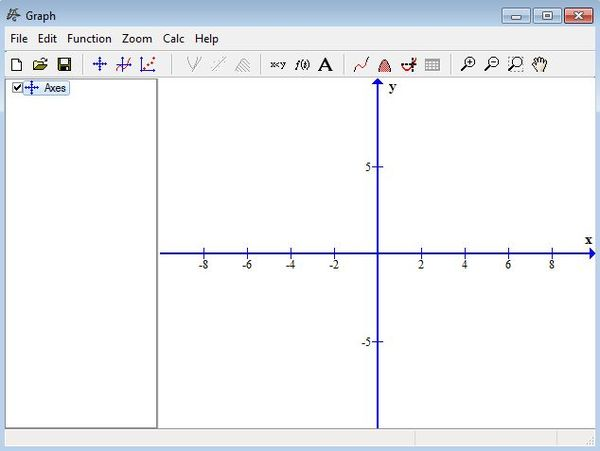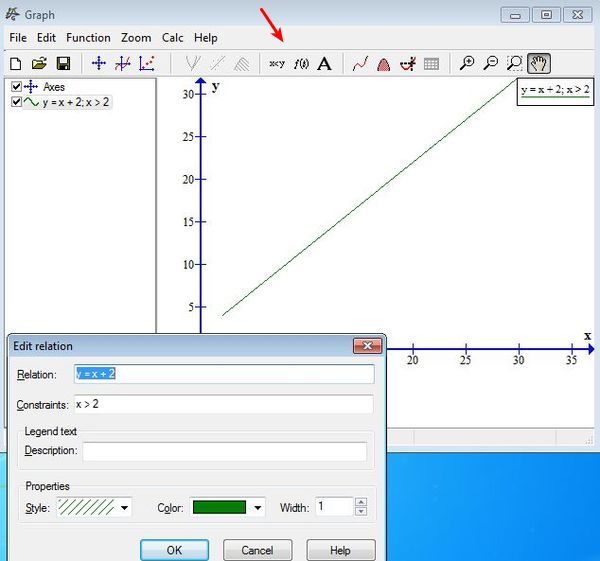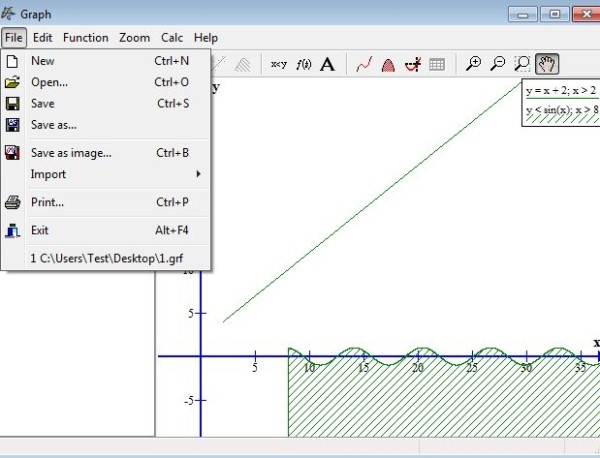Editor Ratings:
User Ratings:
[Total: 0 Average: 0]

Graph is a free mathematical graph drawing software for Windows which you can use in order to draw mathematical graphs in a coordinate system. It can be used in order to draw functions for all the frequently used mathematical functions. Once that you type in functions, the graphs of it are gonna be drawn right away and for every new function that you setup a different mathematical function is gonna be drawn.

Screenshot down below shows us the interface of Graph. You can see on the right a very large and easy to read coordinating system where the math functions that you type are gonna be drawn. Left sidebar will list all the functions that you type. Use the standard menu and the quick access toolbar from the top you can start changing the functions that you want to see drawn.Graphs that you create can be saved in a GIF format, which isn’t an image format, but an editable format that’s used by this free mathematical graph drawing software. Image formats are supported and you can export a graph as image.

## Key features of Graph are:

• Free and simple to use: easily setup math functions and create graphs
• Works with a large number of mathematical functions, trig., linear, etc
• Adjustable scale of the coordinating system: for larger/smaller values
• Zooming: supports zooming in and zooming out sections of the graph
• Supports exporting to both editable and various different image formats
• Supported image formats include: EMF, SVG, BMP, PNG, JPG and PDF
• Printing: generated and created graphs can be printed out
• Works with all versions of Windows, starting with Windows XP

Similar software: yEd Graph Editor, Chart Creator, Functy.

Math functions have to be drawn mostly in schools, and that’s exactly for whom this free mathematical graph drawing software is intended for. Both high school and college students can use Graph, to draw math functions for their assignments. Here’s a few pointers to help you get started.

## How to draw math functions with Graph: free mathematical graph drawing software

The first thing that you’re gonna be needing is a click on the equation or inequality button, so that you can type it in. Small window will pop-up where you can type in the math function that you want to display.Other buttons are available from the toolbar at the top where you can type in even more complex expressions for which then the graph is gonna be drawn.You can add additional functions and then control which are gonna be shown using the left sidebar. When you’re done creating all the graphs that you want, simply click on the File >> Save as image button or File >> Save if you want to save an editable copy of the file.

## Conclusion

Graph does an excellent job when it comes to creating graphs. You might need a minute or two to figure out how to type in the math functions, but if you’re math savvy, then you’re gonna see that everything’s actually very easy to setup and everything works as advertised. If you need to create graphs of math functions in coordinating system for school or projects that you’re working on, this is the tool for you.